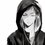$2^{2^{2017}} + 3$ is a prime number or not?Note by Rony Phong
5 years, 11 months ago

This discussion board is a place to discuss our Daily Challenges and the math and science related to those challenges. Explanations are more than just a solution — they should explain the steps and thinking strategies that you used to obtain the solution. Comments should further the discussion of math and science.

When posting on Brilliant:

• Use the emojis to react to an explanation, whether you're congratulating a job well done , or just really confused .
• Ask specific questions about the challenge or the steps in somebody's explanation. Well-posed questions can add a lot to the discussion, but posting "I don't understand!" doesn't help anyone.
• Try to contribute something new to the discussion, whether it is an extension, generalization or other idea related to the challenge.

MarkdownAppears as
*italics* or _italics_ italics
**bold** or __bold__ bold
- bulleted- list
• bulleted
• list
1. numbered2. list
1. numbered
2. list
Note: you must add a full line of space before and after lists for them to show up correctly
paragraph 1paragraph 2

paragraph 1

paragraph 2

[example link](https://brilliant.org)example link
> This is a quote
This is a quote
    # I indented these lines
# 4 spaces, and now they show
# up as a code block.

print "hello world"
# I indented these lines
# 4 spaces, and now they show
# up as a code block.

print "hello world"
MathAppears as
Remember to wrap math in $$ ... $$ or $ ... $ to ensure proper formatting.
2 \times 3 $2 \times 3$
2^{34} $2^{34}$
a_{i-1} $a_{i-1}$
\frac{2}{3} $\frac{2}{3}$
\sqrt{2} $\sqrt{2}$
\sum_{i=1}^3 $\sum_{i=1}^3$
\sin \theta $\sin \theta$
\boxed{123} $\boxed{123}$

Sort by:

SR :)) I just fix it =))

- 5 years, 11 months ago

Nope. Apply mod 7 followed by Fermat's Little Theorem.

- 5 years, 11 months ago

By using Fermat's Little Theorem, we have : $2^{6} \equiv 1 \pmod{7} \Rightarrow 2^{8} = 2 ^{2^{3}} \equiv 4 \pmod{7}$

So that : $2^{2^{4}} \equiv 8 \pmod{7}, 2^{2^{5}} \equiv 6 \pmod{7}, 2^{2^{6}} \equiv 2 \pmod{7}, 2^{2^{7}} \equiv 4 \pmod{7},....$

We can see that if $m = 4 \cdot n - 1$ then $2^{2^{m}} \equiv 4 \pmod{7}$

Thus , $2^{2^{2017}} \equiv 6 \pmod{7}$ ... And I get stuck here :(

- 5 years, 11 months ago

- 5 years, 11 months ago

With tower of exponents, you should be applying Euler's Theorem. This tells us that we should find $2^n \pmod{ \phi(7) }$ first.

Staff - 5 years, 11 months ago

not a prime number

- 5 years, 11 months ago

I think it is prime because $2^{2^{2017}}+1$ is prime and many primes occur at difference of 2, so due to increased probability I chose it to be prime.

- 5 years, 11 months ago

I think $2^{2^{2017}} + 1$ is not a prime because it's is a Fermat number, and until now, there is just 5 Fermat number that are primes is : $2^{2^{0}} + 1; 2^{2^{1}} + 1;2^{2^{2}} + 1;2^{2^{3}} + 1;2^{2^{4}} + 1$

I have prove that $2^{2^{2017}} + 3$ is not a prime number, but thanks anyway.

- 5 years, 11 months ago

No.

Last few decimal digits of the solution are.... ...0172573696 <-Last Digit Even != Prime

Approx Solution:

4530133771486397128616413953101758728033979316264652841195935761051588450033937045213076515129395654998815159098974101615963200558154274787748738790211678644410538970061235159505665699096705221172128501364423224257417606736821962911252833066060363075687976750995313953457727475457383546376473713782226055256851957323635233060085750073840982862112794321584114078317329301232817710611834752430415334301714161673057478502612297701175639541114423640398305254720345616205423349569568002063030321637229012200090760969205506795214577344211530377676944078369371486135407432181252977569490258039805559010498267001268 decimal digits

~~ 4.530133771486397×10^606 decimal digits

- 5 years, 11 months ago

How are you finding the last decimal digit? Are you using a computer? If so, what is the extent of the rounding error?

It is obvious that this number is odd, and hence the last digit is not 6.

Staff - 5 years, 11 months ago